Frequency ModulationNext: Digital Modulation Schemes Up: Analog Modulation Schemes Previous: Amplitude Modulation

## Frequency Modulation

FM is a so called angle modulation scheme, it was inspired by phase modulation but has proved to be more useful partly for its ease of generation and decoding. The main advantages of FM over AM are:

1. Improved signal to noise ratio (about 25dB) w.r.t. to man made interference.
2. Smaller geographical interference between neighboring stations.
4. Well defined service areas for given transmitter power.
1. Much more Bandwidth (as much as 20 times as much).
2. More complicated receiver and transmitter.

In this scheme the frequency of the modulating signal is changed in proportion to the message signal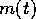. Thus the signal that is transmitted is of the form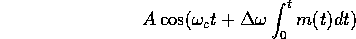Here the signal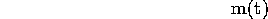is assumed to be normalized so that the maximum of the integral is 1 and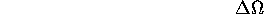is called the frequency deviation of the modulation scheme. The index of modulation of an FM signal of the form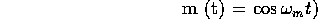is defined to be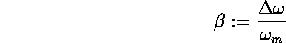Figures 3, 4, and 5 are examples of what FM signals look like in the time domain for a message signal of the form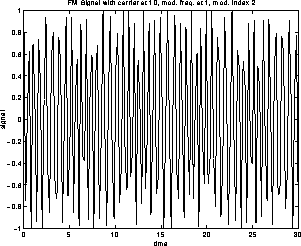Figure 3: FM modulation with modulating frequency 1, carrier frequency 10 and modulation index 2

In general the determination of the frequency content of an FM waveform is complicated, but when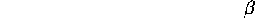is small, for a sinusoidal signal, the bandwidth of the FM signal is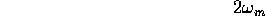On the other hand whenis large, the bandwidth is determined (empirically) to be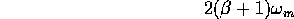Your FM dial goes from 88 MHz to 108 MHz (this range is in between the frequency ranges for the TV channels 1-6, and 7-12. For a typical radio station,varies from about 150 at low frequencies like 50 Hz to 3.75 at high frequencies (20 KHz), and a very rough bandwidth figure is 200 KHz. Thus, KDFC 102.5 on your FM dial goes from 102.4 MHz to 102.6 MHz.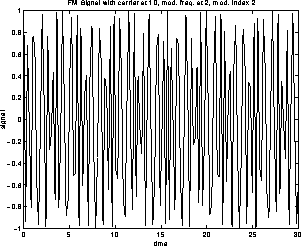Figure 4: FM modulation with modulating frequency 2, carrier frequency 10 and modulation index 2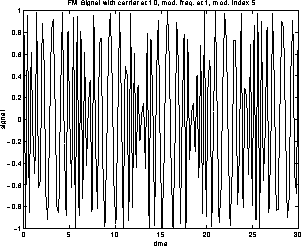Figure 5: FM modulation with modulating frequency 1, carrier frequency 10 and modulation index 5

Try to puzzle over how you might try to demodulate FM (think differentiation!).Next: Digital Modulation Schemes Up: Analog Modulation Schemes Previous: Amplitude Modulation

S Sastry
Sun Apr 20 16:50:27 PDT 1997